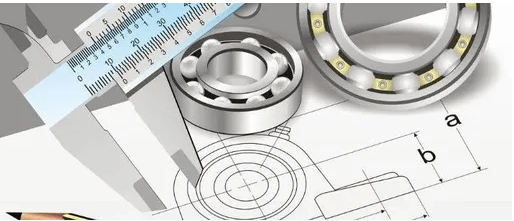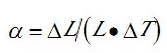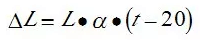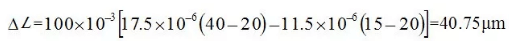# Temperature Influence On Measurement Dimension (Basic Analysis)

We are doing a lot of different measurements every day, and we are pursuing the accuracy of measurement in the process of measurement.

Today, we will talk about the influence of temperature on length measurement.## 1. Factors affecting temperature error

In measurement conditions, temperature, humidity, vibration, dust and corrosive gas can directly or indirectly affect the measurement accuracy.

Among these factors, the change of temperature has a significant effect on the measurement accuracy.

Because the expansion coefficient of different objects is quite different, the principle that the temperature of the measured object is equal to that of the standard instrument is adopted

In order to ensure the accuracy of the test data, all kinds of Metrology specialty should work according to the temperature conditions required by the verification regulation.

At present, the standard temperature of measurement room is 20℃.

According to the manual, the relationship between linear expansion coefficient a and temperature change and size change is as follows, which can be expressed by formula (1):Where:

• a -coefficient of expansion;
• ΔL – size change;
• L – object size;
• ΔT – temperature variation.

The size change of a workpiece due to its temperature deviation from 20℃. It can be expressed by formula (2):Where t is the temperature of the object.

When the temperature of the workpiece and the measuring tool deviates from the standard temperature, the measurement error caused by the temperature is the difference between the two-dimensional changes, and the calculation formula can be expressed as equation (3):Where:

• ΔL is the size change;
• L – object size;
• a1, a2 – linear expansion coefficient of workpiece and measuring tool material;
• t1 t2 -the temperature of the workpiece and the measuring tool.

Definition of linear expansion coefficient of object: when the temperature changes by 1℃, the size change of unit length (1 mm) is only related to the object material.

For example, suppose that the temperature of the copper shaft with diameter of 100 mm is 40℃, the dry micrometer of the outer diameter is 15℃, and the linear expansion coefficients of the copper shaft and the steel micrometer are 17.5 x10-6 and 11.5 x10-6 respectively, then the dimension change △L is:It can be seen that the temperature has a great influence on the size of the workpiece, and there are dozens of errors in this case.

Therefore, when measuring instruments, the temperature error will be greatly reduced if the workpiece and measuring tool are measured after temperature balance.

The temperature and time needed to be balanced in the verification of each measuring tool are specified in the corresponding verification regulations and calibration specifications.

Note: the process that the temperature of the bolted measuring tool and the standard measuring tool tends to be consistent under certain conditions (such as the room temperature is (20 ± 6)℃) is called temperature balance.

## 2. Method of reducing temperature error

1) Measure when the temperature is close to the standard temperature time.

In the actual work, we also strictly follow the relevant regulations, so that customers send measuring instruments and measuring room temperature balance before verification or calibration

So sometimes customers want to get the measurement data immediately, and the measurement data must have temperature effect.

2) In the field measurement, do not take out the standard to measure, but put the standard and the workpiece together, and put it on the large flat plate to measure after isothermal.

3) Avoid the influence of hand temperature on the workpiece and measuring tools.

For example, when using and verifying the micrometer, you must hold the heat insulation pad. When it is found that the hand heat has been transferred to the micrometer, you should place it for a certain period of time in use and verification.

4) In the outdoor measurement of large size, it is necessary to use the measuring instrument to compensate the temperature of the corresponding materials, so as to minimize the temperature error.